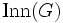# Inner holomorph of a group

(Redirected from Inner holomorph)
Let$G$ be a group. The inner holomorph of$G$ can be defined as the semidirect product$G \rtimes \operatorname{Inn}(G)$ where$\operatorname{Inn}(G)$ is the inner automorphism group with the usual action.
It is a subgroup of the holomorph$G \rtimes \operatorname{Aut}(G)$ and is a quotient of the direct product$G \times G$.
When$G$ is an group having an automorphism whose restriction to the center is the inverse map, this is isomorphic to the central product of two copies of$G$ with the center$Z(G)$ of both copies identified:$G *_{Z(G)} G$.
If$G$ is a group whose center is a direct factor, this group is isomorphic to the direct product of$G$ and$\operatorname{Inn}(G)$.